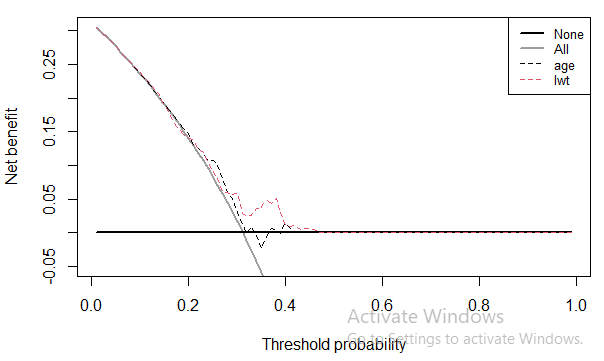# Why the `Treat All` reference line in decision curve is linear?

Hi everyone. I’m interested in NB and Decision Curves and I can’t realize why the treat all reference line is linear.

The general formula is:

NB (general) = (TP / N) - (FP / N) * (p_threshold ) / (1 - p_threshold)

If we are treating all TP / N is equal to ( TP + FN ) / N, Since there are no False Negatives and therefore the expression is equal to the prevalence.

FP / N is equal to (FP + TN ) / N which is infact equal to (1 - prevalence) from the same reason (No True Negatives).

If I understand correctly, the NB of treating all patients is in fact:

NB (treat all) = prevalence - (1 - prevalence) * (p_threshold ) / (1 - p_threshold)

The curve shows the relationship between NB and p_threshold, in the curves the reference line looks like a linear line between (0, prevalence) when p_threshold = 0 and (prevalence, 0) when prevalence / (1 - prevalence) is equal to (p_threshold) / (1 - p_threshold).

If I’m not mistaken, the line must have some curve. Am I wrong?

Thanks!

If you show the full range of the x and y axis, you’ll see the curve. It is pretty linear for parts of the curve. For instance, use the code (this is Stata, but it is similar in R etc):
dca event phat, xstart(.01) xstop(.99) ymin(-10)

3 Likes

Thank you!
The curvy shape is easier to see in the code example provided on decisioncurveanalysis.org:My main goal was to plot to “Interventions Avoided” curve but I’m still confused about it:

I thought it is the difference betwewen NB of the prediction model and the NB of the treating all:

1000 * [NB(model) - NB(all)] / [(p_threshold) / (1 - p_threshold)] =
1000 * (TN/N) - (FN/N) / [(p_threshold) / (1 - p_threshold)]

Obviouusly it’s curly as well. I hope I got it right.

The formula for interventions avoided is given in the original MDM publication and is coded in all the software. It just changes the unit. For a conventional decision curve, we report net benefit in terms of true positives. An NB of 0.1 for a model means e.g. “using the model is the equivalent of 0.1 true positives, that is, it is equivalent to a strategy of biopsying 10% of the population and all of them having cancer, with no unnecessary biopsies (i.e. zero false positives).” If a model had a net benefit 0.1 higher than an alternative model, you can say “using model A rather than model B is the equivalent of finding an extra 10 cancers per patient evaluated without any increase in false positives”.

Now, for interventions avoided, the unit becomes “decrease in false positives” rather than “increase in true positives”. So a model that has 10 per 100 more interventions avoided than treat all, you can say that the model is the equivalent to a strategy where you do 10 fewer biopsies per 100 but find exactly the same amount of cancers.

2 Likes